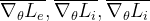# Get grads of parameters w.r.t a loss term in pytorch

I my Pytorch training i use a composite loss function defined as :.
In order to update the weights alpha and beta, i need to compute three values :which are the the means of the gradients of the loss terms w.r.t to all the weights in the network.

Is there an efficient way to write it in pytorch ?

My training code look like :

``````for epoc in range(1, nb_epochs+1):
#init